# Optical Purity and Enantiomeric Excess

Last updated: February 23rd, 2020 |

All About Optical Purity and Enantiomeric Excess

What is a racemic mixture? What is “optically active”, “optically inactive”, and “optically pure”. What do the terms, “l” and “d” mean? What is the formula for optical purity? What is the difference between optical purity and enantiomeric excess? [OK, that one’s quick: they’re the same thing].

In this post we answer all these burning questions, and do some worked examples of optical purity and enantiomeric excess besides.

This post was co-authored with Matt Pierce of Organic Chemistry Solutions.  Ask Matt about scheduling an online tutoring session here.

## 1. Louis Pasteur And The Discovery Of  “Enantiomers”

In our last post on optical rotation, we saw that when Louis Pasteur crystallized a salt of a compound then known as “racemic acid” he discovered that it formed two different types of crystals.

When redissolved in water, one set of crystals rotated plane-polarized light clockwise (dextrorotatory, “to the right”).

The other set of crystals rotated plane-polarized light to exactly the same extent, but counterclockwise (levorotatory, “to the left”).

This was surprising for two reasons.

• First, racemic acid was thought at the time to be a single compound. It turned out to be a 50:50 mixture of two compounds!
• Second, racemic acid did not rotate plane-polarized light: in other words, it was optically inactive. However, each of the two components were themselves optically active: they rotated plane polarized light to equal and opposite extents.

This immediately explained why racemic acid is optically inactive: just like two equal and opposite vectors cancel to give zero, the equal and opposite rotations due to each component cancel each other out.

Pasteur also noticed that the crystal forms were mirror images of each other. Then, in a leap of insight made without knowing the precise structure of the molecule, he then proposed that the molecules themselves were mirror images of each other.  [note 1]

We now know this to be true. We call two molecules that are non-superimposable mirror images of each other, “enantiomers”.

(Molecules that are superimposable mirror images, we just call, “the same molecule”. In chemistry, “∀” is just “A” that’s been rotated)

## 2. A Racemic Mixture Is An Equal Mixture Of Two Enantiomers And Has A Specific Rotation of 0° (Optically Inactive)

The two components of “racemic acid” are (2S,3S)-tartaric acid, which is levorotatory, or (–); and (2R, 3R) tartaric acid, which is dextrotoratory, or (+). They are enantiomers of each other: stereoisomers which are non-superimposable mirror images.

We now use the term “racemic mixture” to refer to a 50:50 mixture of two enantiomers. (“Racemic” comes from the Latin racemus, or “a bunch of grapes”, a nod to the fact that tartaric acid crystals are also known as “wine diamonds”)

So Pasteur’s achievement was the first resolution of a racemic mixture.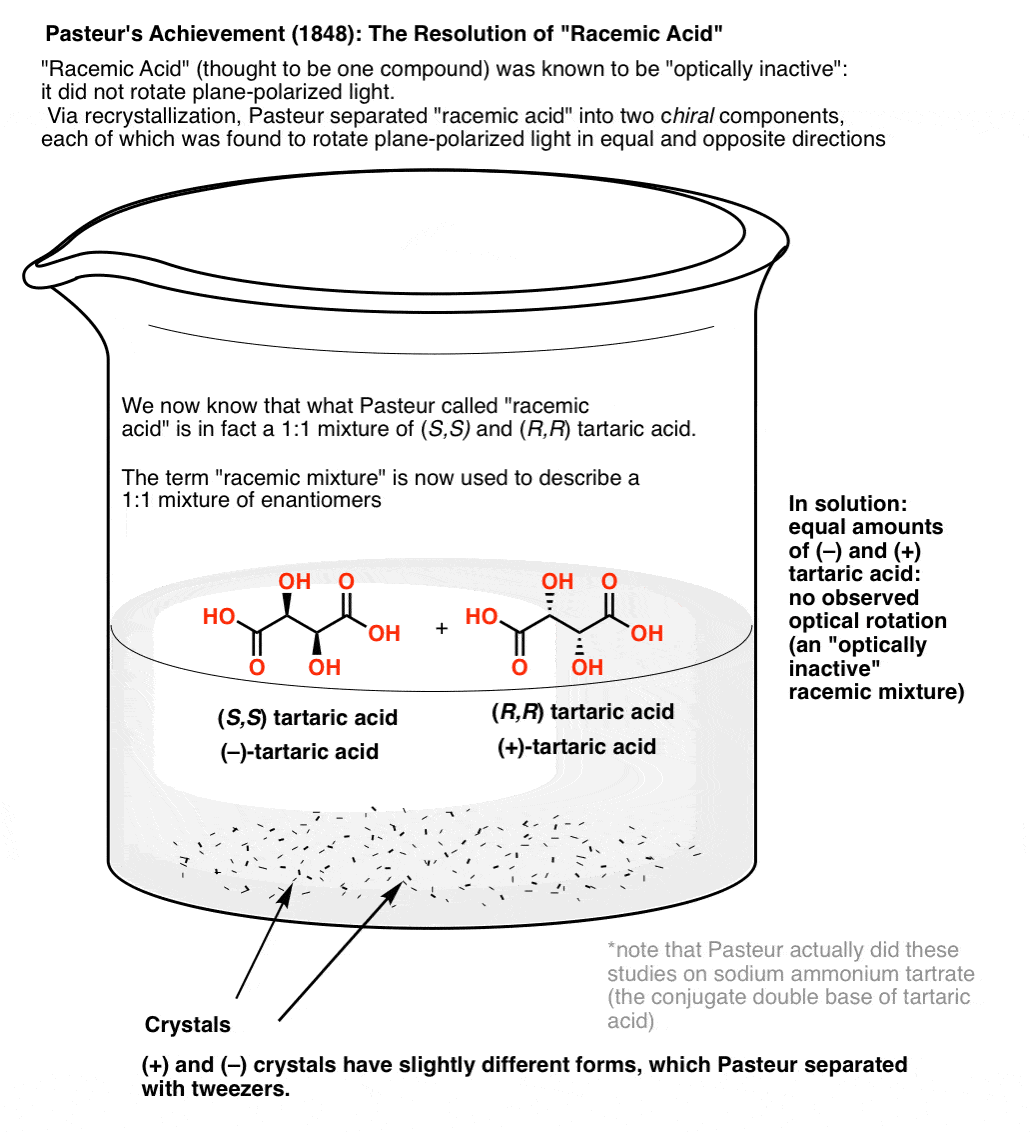## 3. An “Optically Pure” Solution Contains Only One Enantiomer

As we said, the two enantiomers of tartaric acid rotate plane-polarized light to equal and opposite extents. In the last post we introduced a more precise way of saying this, in that the two enantiomers of tartaric acid have equal and opposite specific rotations.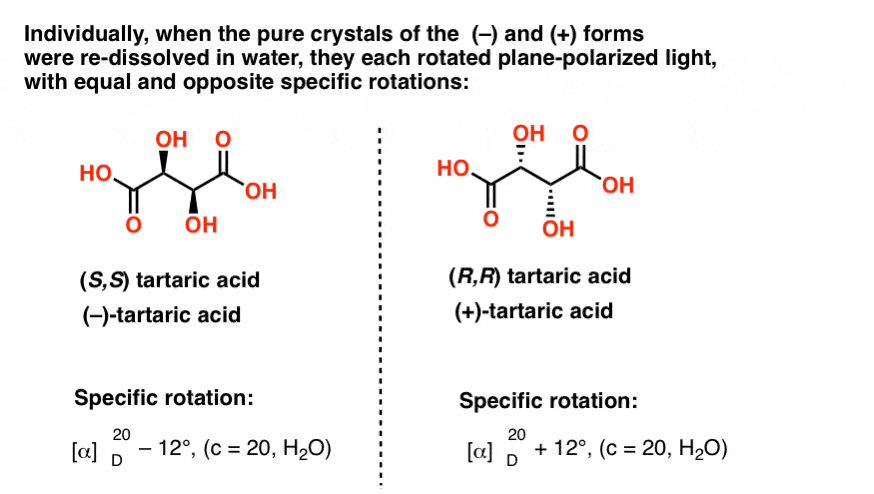These “specific rotations” have to be measured experimentally: there’s no simple correlation between the configuration of a chiral centre (R or S) and the optical rotation.

This term “specific rotation” means that for the given concentration, temperature, wavelength, and solvent

• An “optically pure” (or “enantiomerically pure”) solution of 100% (S,S) tartaric acid and 0% (R,R) tartaric acid will have an optical rotation of –12°.
• An “optically pure” solution of 100% (R,R) tartaric acid and 0% (S,S) tartaric acid will have an optical rotation of +12° .

We’ve also seen that a “racemic mixture” of 50% (S,S) tartaric acid and 50% (R,R) tartaric acid will have an optical rotation of 0°.

## 4. The Terms “l-” And “d-” Refer To The “Levorotatory (–)” And “Dextrorotatory (+)” Forms Of Each Enantiomer

If we call the “levorotatory” (–) form of tartaric acid  “l-”  and the “dextrorotatory” (+) form of tartaric acid “d-“, then we can make the following three points on a graph. [note: in the real world, this graph is not always so linear, but it will do for our purposes]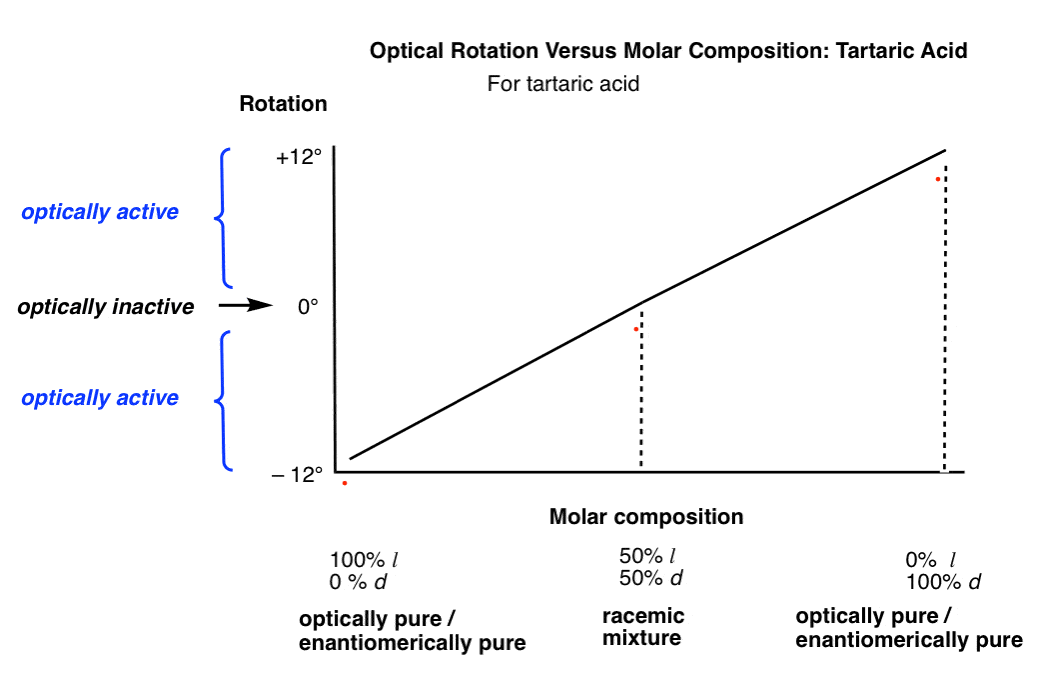100% optically pure l-tartaric acid has a specific rotation of –12°, which gradually rises to 0° as the proportion of the d enantiomer is raised to 50%. As the the molar composition of the d form increases, so does the optical rotation, going up to +12° again for the optically pure d-enantiomer.

## 5. The Formula For Optical Purity

This suggests an idea. Let’s say you had a non-racemic but optically impure mixture of l and d enantiomers, a situation that arises quite frequently in modern organic chemistry .

By measuring the optical rotation of the mixture for a compound whose specific rotation is known,  you can figure out its optical purity and could work backwards to determine the ratio of enantiomers. 

For example, let’s say you had a mixture of enantiomers of tartaric acid that has an observed rotation of +6° .

We can define the optical purity as the observed rotation of a mixture divided by the specific rotation of the pure enantiomer (obtained under identical conditions).For our solution of tartaric acid with an observed rotation of +6°, our optical purity would be 50%## 6. Going From “Optical Purity” To Determining The Molar Composition Of The Mixture

Now comes the part which sometimes trips people up. What’s the molar composition of the mixture?

Your first answer might be 50:50.  50% d- and 50% l- .

But when you think about it, that can’t be right: a 50-50 mixture would be racemic, which would have no optical rotation at all!

An “optical purity” of 50% actually means that the major enantiomer (d- in this case, since the solution is dextrorotatory) is present in 50% excess over the minor enantiomer. (l-)

The molar composition of the l-  and d- forms must add up to 100%: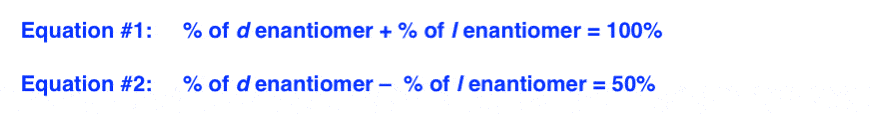So using some basic algebra, we can use these two equations to calculate the ratio of enantiomers.

For instance, in equation #2,  we can isolate the % of d- as being (50% + % l- ). We can then plug that value into equation #1 and we obtain the value for % l-  as 25%. The value for % d- must then be 75%.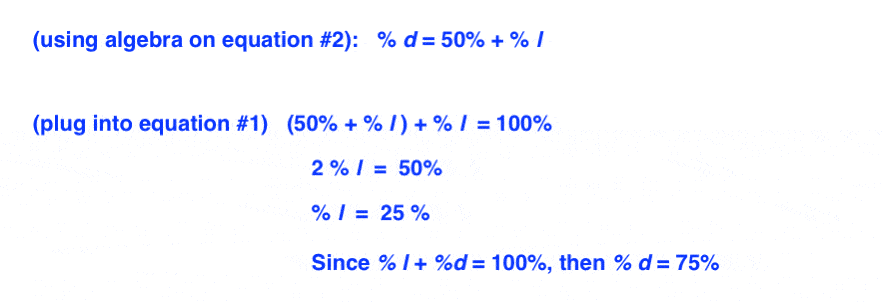So, far from being 50-50,  a mixture with 50% optical purity is actually a 75% : 25% mixture of enantiomers.

One way of looking at a 50% value for “optical purity” is that it’s 50% the dominant enantiomer, and 50% a racemic mixture.

## 7. Optical Purity = Enantiomeric Excess

If you do the math in the above example,  you’ll note that the percentage of the d– enantiomer (75%) exceeds the percentage of the l- enantiomer (25%) by 50%. This also happens to be the value we calculated for optical purity.

Thus, the optical purity is thus equal to the percentage excess of the major enantiomer over the minor enantiomer.

This term, the “enantiomeric excess”, or “e.e.” for short,  is equivalent to the optical purity and is actually used far more often for expressing the enantiomeric purity of a mixture.

So an “optically pure” solution containing 100% of the d – enantiomer and 0% of the l- enantiomer would have an enantiomeric excess of:

100% – 0% = 100%

The same would be true for an optically pure solution of the l- enantiomer: 100% e.e.

A racemic mixture, as we saw, has an optical purity of 0%. And so, too,  the enantiomeric excess is also 0%.

50%d  – 50% = 0%

## 8. Another Practice Problem For Enantiomeric Excess

Let’s do another problem!

A sample of 2-bromobutane has an enantiomeric excess (e.e.) of 75% favouring the d– enantiomer. What is the percentage of each enantiomer?

First, identify the relevant equations:

e.e. = %d – %l  = 75%

%d- + %l-  = 100%

Next, solve one equation for either variable so you can plug that into the other equation. In this case we arbitrarily solve for the % of the d– enantiomer using the second equation.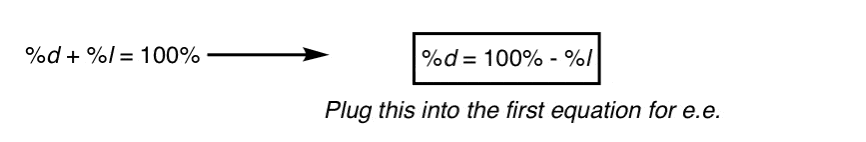Now, plug in what we found to the first equation, solving in this case for the percent of the d- enantiomer.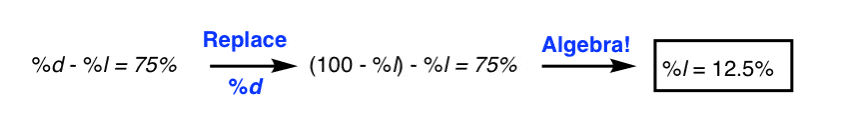Then, solve for %d with our knowledge that the total equals 100%.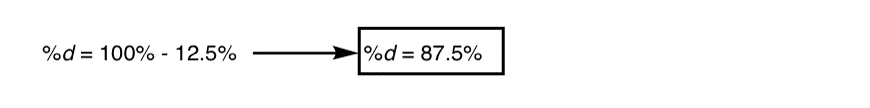The previous two steps contain the answer, but we can also double check by plugging these numbers into the equation for e.e.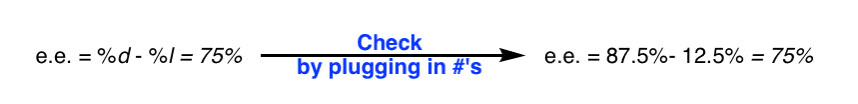So a mixture with 75% ee is actually composed of 87.5% of the major enantiomer, and 12.5% of the minor enantiomer.

As we said above, another way of looking at it is that it’s 75% composed of the major enantiomer, and 25% of a racemic mixture.

## 9. An Optical Purity Practice Problem

Let’s do a slightly different problem, this time using optical purity.

A mixture contains 3g of (+)-2-bromobutane and 2g of (–)-2-bromobutane. What is the rotation of the mixture, given that (+)-2-bromobutane has a specific rotation of +23.1°.

First, let’s calculate enantiomeric excess/optical purity as a decimal number. Note that the units here (grams) will cancel.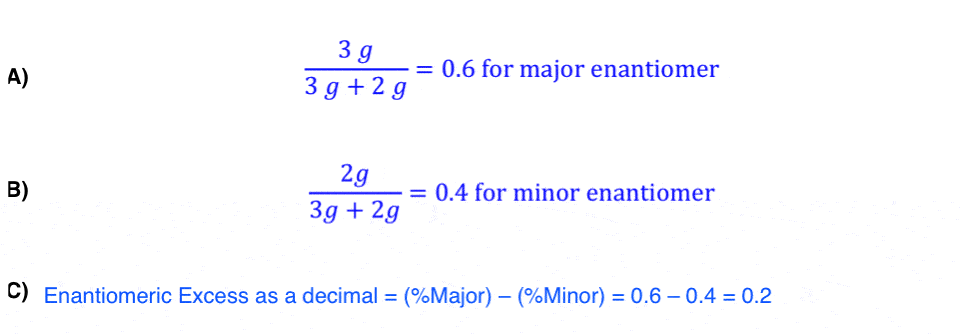Now, we’ll solve for the rotation of the mixture using the equation for optical purity. Again, we’ll use the decimal form, which is 0.2 , for optical purity (or enantiomeric excess).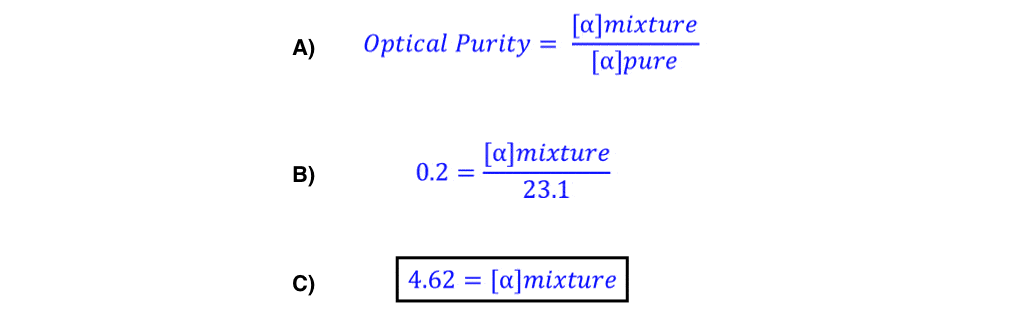This gives us a rotation of 4.62° for the mixture.

That’s it! By the way, this is about as intense as math will ever get in introductory organic chemistry. You’re welcome.

This ends our two part discussion of optical activity, polarimetry, specific rotation, and optical purity.

Thanks again to Matt for helping with this post. Hire Matt as your tutor!

## Notes

[Note 1] This was proposed in 1848, about 25 years before Van’t Hoff proposed tetrahedral carbon. Pasteur’s work was one of the foundations on which Van’t Hoff made his proposal; a carbon with 4 different groups can only exist as two different enantiomers if the carbon is tetrahedral. It’s impossible if carbon is square planar.

[Note 2] These situations frequently come about when using an enantioselective reaction on an achiral compound, such as the epoxidation reaction that won Barry Sharpless the 2001 Nobel Prize (fittingly, this reaction employs the enantiomers of tartaric acid to impart  “handedness” to the produchttps://pubs.rsc.org/en/content/articlelanding/2018/sc/c7sc04249c#!divAbstractts).  Enzymes are likely a more familiar category of enantioselective catalysts: Baker’s yeast has been used for the enantioselective reduction of ketones, for instance.

[Note 3] Optical rotation measurements (i.e. polarimetry) are actually an unreliable way to determine enantiomeric excess. There are three reasons.

• The optical rotation of the pure enantiomers may not be known
• Small amount of optically active impurities may cause the experiment to give drastically incorrect results [UPDATE: Like in the real-life example of Frondisin B].
• Optical rotation doesn’t always have a linear relationship to enantiomeric excess like we’re claiming it does here. This is because of the Horeau Effect.

A more common way to measure enantiomeric excess is through HPLC or GC with a chiral stationary phase, or through the Mosher method.

## Comment section

### 5 thoughts on “Optical Purity and Enantiomeric Excess”

1.sahani philipo says:

problem in identifying the compound which is in excess in the racemic mixture when the %ee is 26.38, in such away that when you some the racemate do not give 100% help pls im in trouble with this confusion.

2.Dr.R.Vijayalakshmi says:

By simply adding equation 1 and 2, one can get % of d enantiomer = 150/2 =75. Substituting this in any one of the equations will give % of d enantiomer to be 25.

3.lontum rodrique says:

sir, still confuse in the above explanation whether ee is 25 or 75

4.Dr. Swapnil Patil says:

Hi James,
I always admire your website, in fact, I do recommend this to my students as well.
About the “The Formula For Optical Purity” you have mentioned
Optical purity or % ee = |observed rotation| / |specific rotation of pure|
However, I think it may be:
Optical purity or % ee = |observed specific rotation| / |specific rotation of pure|
Some times students may get confused with observed rotation and observed specific rotation.

1.James Ashenhurst says:

Yes, thank you – that will be fixed shortly.

This site uses Akismet to reduce spam. Learn how your comment data is processed.